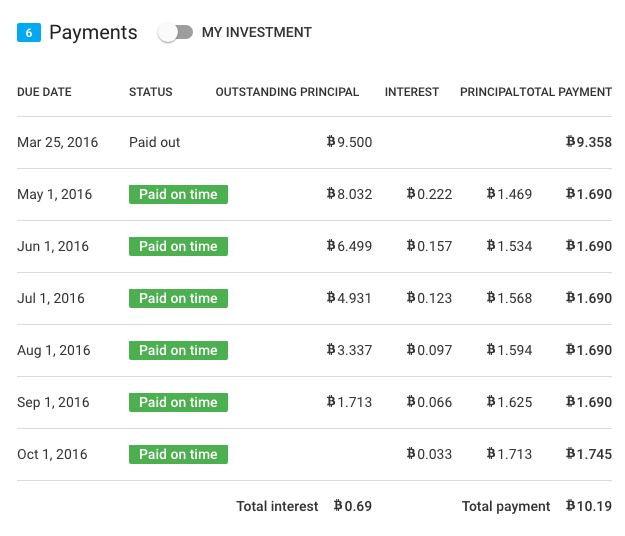# How are interest payments calculated?

The interest rate is one of the most important aspects of a loan. For the borrower the interest rate represents the cost of borrowing. For the lender the interest rate is usually one of the main reasons to invest. Different loans have different interest rates and we want to show you how they are calculated.

## Nominal interest rate

The nominal interest rate is the rate that we use in order to  calculate interest payments on a loan. The nominal interest rate is always stated as an annual (or per annum – p.a.) rate. This is because the duration varies from loan to loan. If we did not state an annualized rate it would be very difficult to compare the interest rates of different loans. The annualized representation of interest rates is also called annual percentage rate (APR).

When an interest payment is calculated, we consider the duration over which the funds have been borrowed and take compound interest into account.

### Example calculation for a 6 week loan

The easiest example is our 6 week loan. This loan has no installments. It is paid out and at the end of the 6 week loan duration the principal plus the interest payment is due. The payment is calculated as follows:

• Loan amount x EXP(interest rate x 6 weeks / 1 year) where EXP() stands for the mathematical constant e

Let’s calculate a concrete example. The loan amount is BTC 0.5 and the nominal interest rate is 13% p.a.

• 0.5 BTC x EXP(13% x 42/365)
• 0.5 BTC x 1.01507135 = 0.50753567
• The interest payment is therefore 0.50753567 – 0.5 = 0.00753567

Since the bitcoins were borrowed only for 6 weeks (which equals 42 days) we have to make sure that this is the basis for the interest calculation. This is done with the 42/365 part. If we simply calculated 0.5 BTC x EXP(13%) the result would correspond to a one year lean. The interest payment would be overstated.

### Example calculation for a 6 months loan

The interest calculation on an amortizing loan has to take into account that the borrowed amount is reduced every month by the principal repayment. Therefore the interest portion of each payment also varies. Interest is always calculated based on the outstanding principal of the previous month. Let’s look at an example payment schedule:If we take payment no 3 as an example which is due on July 1st the following calculation applies:

• Outstanding principal at the payment due date x (EXP(interest rate x month of June / one year) - 1)
• 6.499 BTC x (EXP(22.74% x 30 days / 366 days) -1)
• 6.499 BTC x  0.018814142 = 0.122273

If the loan was paid on a say weekly basis the same logic would apply. The only difference would be that instead of 31 days we would need to plug in 7 days. However, even in the case of weekly payments we would state the interest rate on an annualized basis. Only then we can compare the relative cost / the relative return of this loan with other loans.

## Effective interest rate

The effective interest rate, also called  internal rate of return (IRR), can differ from the nominal rate. In case of the bitcoin loans originated via Bitbond this especially applies to the borrower. The effective interest rate has purely for informational purposes. The calculation of actual interest payments is always based on the nominal interest rate as stated above.

### Borrower perspective

The  borrower pays a fee which is a percentage of the loan amount. We have said at the beginning of this blog post that the interest is the cost of borrowing. Since the fee increases the cost of borrowing to the borrower it should be included in the effective interest rate of a loan.

The disbursement to the borrower is always made net of the origination fee. For the above loan it would therefore not be BTC 1.0 (the loan amount) but BTC 0.995 (because the origination fee on loans with a 6 months term is 0.5%).

If we calculate the IRR on the cash flows in the ‘Total payment’ column where payment no 0 is BTC 0.995 we get a value of 29.78%. This is the effective interest rate to the borrower. It considers all cost (interest and fee payments) that the borrower has to bear.

### Lender perspective

To the lender the effective interest rate also differs from the nominal rate. If you calculate the IRR of the ‘Total payment’ column the result is 27.86% which is 3.28%-points more than the nominal interest rate. This is because the IRR calculates the theoretical rate that is necessary to make all cash flows equal to the initial payment. Over longer time horizons the IRR typically grows versus the nominal interest rate.